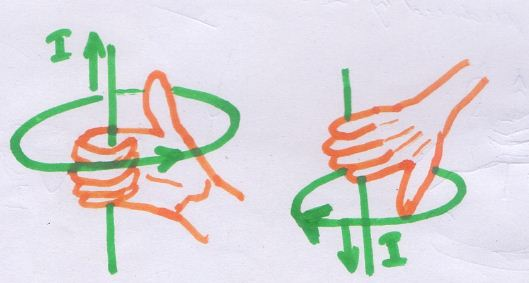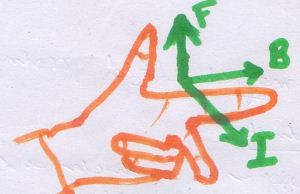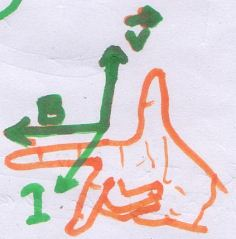# PhysicsTeacher.in

## High School Physics BLOG - online class notesRSS Feed

# Electromagnetics: Fleming’s Left hand Rule, Right Hand Rule, Maxwell’s Right hand grip rule

Electromagnetic Force manifests itself in 2 avatars, Electricity and Magnetism. Electromagnetism is a branch of physics that describes the interactions of electricity and magnetism. A magnetic field is created by a moving charges i.e. electric current. Again a magnetic field can induce movement of charges (electric current). Magnetic Field and a conductor (may be current carrying or not carrying, may be static or moving) can have different mutual effects in different scenarios, based on some specific conditions. Let’s find out a few such incidents of electromagnetism and in the process let’s discuss on few Laws like Right hand thumb rule or right hand grip rule, Fleming’s Left hand rule and Right hand rule. We also figure out motor law and dynamo law (or generator law).

A magnet produces a magnetic field in the space around it. This field exerts a force on any other magnet placed in that field. Each point in this field has a particular strength (field strength) and a definite direction.When we keep a magnetic compass in the field of a magnet (say a Bar magnet), the direction of its needle gives the direction of the net magnetic field due to the bar magnet and the earth’s magnetic field. However, if we keep the compass very close to the magnet, the earth’s field may be neglected.

Great! So generation of magnetic field can be credited to a magnet! Now we also know that a magnetic field can also be created by an electric current in a conductor. Danish Physicist H.C. Oersted noted this first in 1820.

## Magnetic Field created by a straight current carrying conductor – RIGHT HAND THUMB RULE of Maxwell

The magnetic effect of electric current can also be easily shown by placing a magnetic compass near a wire which is carrying electric current. We see that the needle of the compass is deflecting, which proves that a magnetic field is produced near the wire.

The direction of the magnetic field due to a current carrying conductor: If we consider a straight conductor which is carrying current, then the direction of magnetic field created can be found using the RIGHT HAND THUMB RULE of Maxwell.

### Cause and Effect:

So RIGHT HAND THUMB RULE of Maxwell helps to find the direction of the magnetic field produced by a straight current carrying conductor. Here cause is the current that flows through a straight conductor and the result is the creation of a magnetic field.Maxwell Right hand thumb or grip rule

In the diagrams above, I is pointing towards the direction of the current. The Circle with arrow direction-pointer indicates the direction of the magnetic fields.

### RIGHT HAND THUMB RULE of Maxwell – statement

The rule says, if we imagine holding a straight current-carrying wire in the right hand, with the thumb stretched along the direction of the current, then the direction in which the other fingers are curled will point the direction of the magnetic field created by the current carrying conductor.

This is also known as RIGHT HAND GRIP Rule.

## Effect of a Magnetic Field on a Current Carrying Conductor- Fleming’s Left Hand Rule

Now if we think in the opposite way, i.e. a magnetic field is already present and a current carrying conductor is brought in the magnetic field.

### Cause and Effect:

In other words, here cause is the magnetic field already present and the result medium is the current carrying conductor. And the result or effect is a force generated which acts on the current carrying conductor and deflects it.

An experimental setup can be prepared using a horse shoe magnet (or any other type of magnet) and a thick copper wire connected to a battery via a switch. Here we can find that the current carrying conductor wire deflects due to a force generated by the magnetic field of the magnet present nearby.

Here comes another set of rule called ‘Fleming’s Left hand rule’, which helps us to find out the direction of the force experienced by the current carrying conductor placed in  a magnetic field that is perpendicular to the current flow direction in the wire.

(Note this: it’s applicable only if conductor is placed at right angle (perpendicular) with respect to the direction of the magnetic field present.)Fleming’s Left Hand Rule

In the diagram above, B, I and F are pointing towards the directions of magnetic field, Current through the conductor and the force experienced by the conductor RESPECTIVELY.

### Left hand rule of Fleming – statement

If the fore finger, second finger and thumb of the left hand are stretched at right angles to each other, with the forefinger in the direction of the magnetic field, and the second finger in the direction of the current, then the thumb indicates the direction of the force acting on the current carrying conductor.

This Left hand Rule is also known as Motor Rule. Experiment related to this rule – Barlow’s wheel

## Effect of a Magnetic Field on a moving Conductor – Fleming’s Right Hand Rule

Now if we modify the previous setup a bit and do the following:

– instead of placing a current carrying conductor in a magnetic field, we will place just a conductor initially, without any current flowing through it. So just a conductor is what is required to be in the magnetic field and yes, it’s to be placed at right angle to the direction of the magnetic field present; no need of any battery and switch.

– This time the conductor has to be moved, i.e. the conductor is not static, and it’s moving in the magnetic field.

So are you getting the difference of this setup with the previous one? The previous one dealt with a conductor which is already carrying a current. When it’s placed in a magnetic field at right angle, then it would result a deflection or movement in the conductor.

But in this case, no current needs to flow initially through the conductor. And this time the conductor is given a movement at right angle to the magnetic field. (This is the setup required)

Do you know what the result is this time?

This setup caused generation of an electromotive force in the conductor which results into an induced flow of current through the moving conductor.

### Cause and Effect:

So here cause is the movement of a conductor in a magnetic field at right angle and the result is generation of current flow through the moving conductor.

Fleming’s right hand rule comes handy here.Fleming’s Right Hand Rule

In the diagram above, B indicates magnetic field, I indicates the induced current and V indicates the movement of the conductor in the magnetic field.

### Fleming’s Right Hand Rule – statement

If the fore finger, second finger and thumb of the right hand are stretched at right angles to each other, with the forefinger in the direction of the magnetic field and the thumb in the direction of the movement of the conductor then the second finger will indicate the direction of the current induced in the moving conductor.

This Rule is also known as Dynamo or Generator rule.

#### Interesting Posts:

Electric Charge

generator effect and motor effect – principle

Free Fall versus Drag Force – Terminal Velocity
Thermal Conductivity

Suggested site

error: Content is protected !!